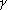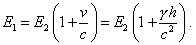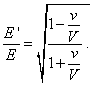SELF 4 S.B. Karavashkin and O.N. KaravashkinaIn distinct from an accelerated reference frame, if the frame falls freely in a gravity field, all bodies in this frame will be equally accelerated, so they will behave as the quasi-inertial. In this reference frame, the momentum and energy conservation laws will be true in limits of accompanying reference frame (although in narrow limits, and we have always to account it and to check). These laws will be violated as to physical processes which gravity acceleration does not affect. And Einstein agrees that "we can say nothing a priori about the energy transfer by radiation, since we do not know, how the gravity field affects the radiation energy and the measuring instruments in S1 and S2 " [16, p. 168]. None the less, it cannot stop Einstein in his statement, "until we confine ourselves to mere mechanical phenomena, for which Newtonian mechanics is true, we are sure (? - Authors) that the frames K and K' are equivalent. But our representation will be enough deep only if the frames K and K' are equivalent relatively to all physical phenomena, i.e. if the laws of nature in relation to the frame K fully coincide with the laws of nature in relation to the frame K'. Having admitted it, we yield the principle of great heuristic importance, if it is really true" [16, p. 166- 167]. Important addition: if it is really true! Henceforth this addition will disappear from the conditions of applicability of frames equivalency postulated by Einstein, as well as will disappear even mentioning that (4), determining the light velocity variation with respect to gravity potential, might be correct only in the first order of approach and only to a narrow amount of trajectories. As we mentioned before, the body freely falling in a gravity field, has a very limited virtual inertiality. If we consider a body freely falling in a gravity field and having no transverse speeds, this body will really remain at rest relatively the reference frame freely falling in this field. It can seem, this body looks like an inertial body in absence of gravity. But if we add to this body some motion transverse to the free fall, our analogy will be destroyed: in frames of Newtonian gravity paradigm, in the view of inertial reference frame, it would be simple to show that in the non-inertial reference frame, the body's trajectory already will not be direct (we will show it in the item 6). These deviations from inertiality, with violated conservation laws typical for non-inertial reference frame, are conditioned by variations of the angle of gravity force affection as to the axis of inertial reference frame during the transverse shift of the studied body. Thus, the laws that, after Einstein's thought, would have to be equally true both in inertial reference frame and non-inertial reference frame, factually cannot be true in general case. Just as in case of SR postulates formulation on a limited gamut of motions, in formulating the equivalency principle, the meaning of this principle can be applicable to a very narrow gamut of motions in non-inertial reference frames that disables us to think this identification not only as that general, inherent in all laws of nature, but even as some local, true under a quasi-permanent potential in the studied area. Undoubtedly, on such 'reliable' basis one can substantiate any thesis, in that number growing velocity of emission in an accelerated reference frame: "Let us be discussing the energy transfer by way of radiation from S2 to S1, being located in some non-accelerated reference frame K0. Let us think that at the moment when the radiation energy E2 is transmitted from S2 to S1, the frame K'  has a zero speed relatively the frame K0. … At this moment, the speed of S1 relatively K0 ish/c =. So, according to a trivial relativity theory, the energy of radiation that achieves S1 is not E2 but larger energy E1 which in the first approximation is interrelated with E2 as(10)
 In our supposition, exactly the same relationship is true also in case when the considered process takes place in a frame K, which is non-accelerated but located in the gravity field" [16, p. 168]. Firstly, it follows from this citation that from the view of stationary observer located in K0 , the energy received by the receiver in S1 grows only because the receiver moves. Secondly, in the formula for energy transformation to which Einstein refers [20, p. 28], the relation is slightly other:(11)
 And there Einstein emphasises so: "It is significant that both the energy and frequency of the light complex vary with the change of observer's state of motion after one and the same law" [20, p. 28]. Thus, Einstein had no grounds to apply to non-inertial reference frames the regularities yielded for inertial reference frames. With it, he ignores the fact that the statements of problems are irrelevant and solutions as well, and the fact that Einstein himself does not know, how the gravity affects the electromagnetic wave, and that the passing from (11) to (10), accurate to the opposite sign (what is the difference - increased, decreased?), is true only at small speeds of a body. It is seen in this all, he based his derivation not on the rigour of substantiation but on his striving to prove by any price that the energy of radiation emitted along the attraction toward the gravitating body has to increase. Naturally, this causes many questions, one more interesting than other. But the very simple question, which fully annihilates all Einstein's substantiation, is - to compare the initial formula (10) and final formula (7) of Einsteinian derivation. Actually, if in the reference frame K0 the light velocity changes relatively to the observer, which velocity Einstein puts to (10)? And the main, on which grounds, if the main postulate of SR - constancy of the light velocity in all reference frames (K0 is claimed to be inertial) - automatically appears untrue? On which grounds he may even approximately use the formula (11) of SR in the problem in which the basis of SR has been violated? Neither Einstein answered such questions of Abraham nor all following generations of relativists will not. They only, after Einstein, will delete from the above derivation his mentioning that it was very approximative, and will use the formula of light transformation (7) as exact and undoubtedly true. Such is, so to say, phenomenology and such, if we may say so, mathematics has been put into the underpinning of Einstein's GR. With it, manipulations with phenomenology and mathematics are not over at this point. Further they will appear even more irresponsible, since clear and peremptory lie put to the statement of problem will again and again remember itself in absurdity of solutions. This will require new and new manipulations. We will show them below, when analysing Schwarzschild's solutions being the basis for the conventional theory of so-called black holes.

Contents: / 1 / 2 / 3 / 4 / 5 / 6 / 7 / 8 / 9 / 10 / 11 / 12 / 13 / 14 / 15 / 16 / 17 /